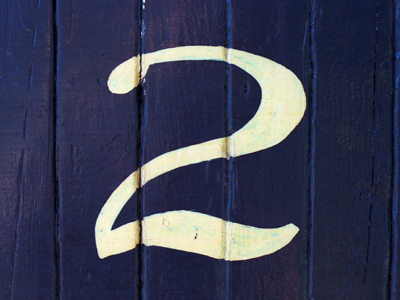If you can count in twos it will help you to multiply and divide by two as well.

# Multiplying and Dividing by 2

This Math quiz is called 'Multiplying and Dividing by 2' and it has been written by teachers to help you if you are studying the subject at elementary school. Playing educational quizzes is an enjoyable way to learn if you are in the 3rd, 4th or 5th grade - aged 8 to 11.

It costs only \$12.50 per month to play this quiz and over 3,500 others that help you with your school work. You can subscribe on the page at Join Us

Counting in 2s is the earliest multiplication skill taught which also helps children when dividing. It is usually practiced regularly, in order for the skill to be fluent, and children are also taught to recognize that multiples of two are even. Once the 2 times table is familiar, children will learn how to use the multiplication facts to solve division questions. For example, if they know that 2 x 6 = 12, then they may also recognize that 12 ÷ 6 = 2 or 12 ÷ 2 = 6

Use this quiz to practice multiplying and dividing by 2.

1.
What is 20 x 2?
22
40
10
12
Multiplying by 2 is the same as doubling a number
2.
Which is the odd fact out?
6 x 2 = 12
12 ÷ 6 = 2
12 x 2 = 6
12 ÷ 2 = 6
12 x 2 = 24 not 6!
3.
10
25
50
12
5 x 2 = 10
4.
I put 20 children into pairs. How many pairs can I make?
2, because 20 ÷ 10 = 2
5, because 20 ÷ 10 = 5
20, because 20 ÷ 2 = 20
10, because 20 ÷ 2 = 10
We also know that 10 x 2 = 20
5.
How many 2s are there in 28?
16
14
10
8
Counting up in 2s until you reach 28 is helpful, as is halving 20 and halving 8
6.
Is 45 in the 2 times table?
No, because it is an odd number
Yes, because it is an even number
Yes, because it has a 4 at the beginning
No, because it is too big
Multiples of 2 end with 2, 4, 6, 8 or 0
7.
Doubling is the same as...
dividing by 2
halving
subtracting over and over
To double 10, you would add 10 to itself: 10 + 10 = 20
8.
What is half of 60?
2
20
3
30
Finding half is the same as dividing by 2. Knowing 6 ÷ 2 = 3 helps with 60 ÷ 2 = 30
9.
I know that 2 x 15 = 30. What else do I know?
30 x 2 = 15
2 x 15 = 20
30 x 15 = 2
30 ÷ 15 = 2
If 2 groups of 15 makes 30, then 30 divided into 15 groups will mean 2 in each group
10.
What is the next number in this sequence: 2, 4, 6, 8, 10, ?
14
11
12
20
The numbers are going up in twos
Author:  Angela Smith